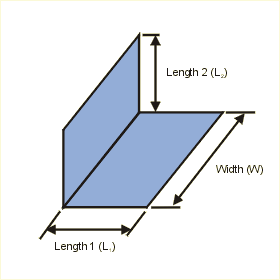Close

This page calculates the net radiant heat exchange for two isothermal (constant temperature) parallel flat black plates of equal size.The heat flow (q) from the plate is calculated as:

q = 56.69x10-9 x VF(1-2) x L1 x W  (T14 - T24)

Where 56.69x10-9 is the average Stefan-Bolzmann constant, L1 is the length of plate 1, W the common width of both plates, T1 is the plate 1 temperature and T2 is the plate 2 temperature. The view factor VF(1-2) (also known as radiation shape factor, angle factor, and configuration factor) is defined as:Where x, y, and z are defined as:

x = L1 / W
y = L2 / W
z = x2 + y2

Where L1 is the plate 1 length, L2 is the plate 2 length, and W is the width of the plates.

References

Ellison G. N., Thermal Computations for Electronic Equipment, Van Nostrand Reinhold Company, New York, 1984. p. 78 - 80.

Holman, J.P., Heat Transfer, 7th ed., McGraw Hill Book Company, New York, 1990. p. 385 - 405.